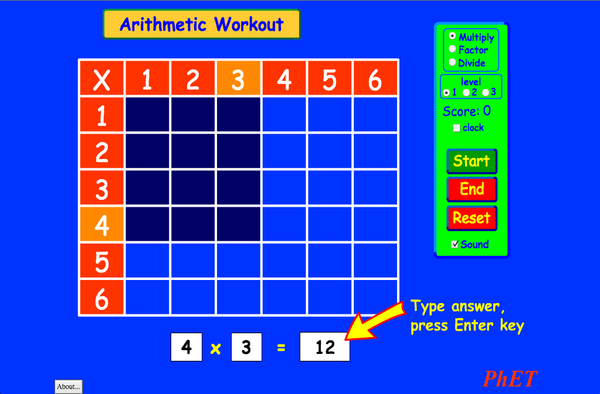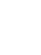# AritmetikaPreuzimanjeUgrađivanje zatvorite Ugradite interaktivnu verziju ove simulacije Koristite ovaj HTML kod da ugradite interaktivnu verziju ove simulacije. Možete promijeniti širinu i visinu ugrađene simulacije tako što ćete doraditi "width"(širina) i "height" (visina) atribute u HTML kodu. Ugradite sliku koja će na klik pokrenuti simulaciju
Kliknite za pokretanje
Koristite ovaj HTML kod da biste prikazali sliku ekrana sa riječima "Kliknite za pokretanje". Množenje PhET je podržan odi učitelja poput Vas.Nazad na HTML5 verziju

• Množenje

### Opis

Remember your multiplication tables? Practice your multiplication, division, and factoring skills with this exciting game. No calculators allowed!

### Uzorak ciljeva učenja

• Explain how multiplication tables help understand multiplication, factoring, and division.
• Use an array model to understand multiplication, factoring, and division.
• Increase accuracy in multiplying, factoring and dividing.
• Develop multiple strategies for arithmetic problems.

### Usklađivanje standarda

#### Zajednička jezgra - Matematika

3.OA.A.1
Interpret products of whole numbers, e.g., interpret 5 × 7 as the total number of objects in 5 groups of 7 objects each. For example, describe a context in which a total number of objects can be expressed as 5 × 7.
3.OA.A.2
Interpret whole-number quotients of whole numbers, e.g., interpret 56 ÷ 8 as the number of objects in each share when 56 objects are partitioned equally into 8 shares, or as a number of shares when 56 objects are partitioned into equal shares of 8 objects each. For example, describe a context in which a number of shares or a number of groups can be expressed as 56 ÷ 8.
3.OA.A.4
Determine the unknown whole number in a multiplication or division equation relating three whole numbers. For example, determine the unknown number that makes the equation true in each of the equations 8 × ? = 48, 5 = _ ÷ 3, 6 × 6 = ?
3.OA.B.5
Apply properties of operations as strategies to multiply and divide.2 Examples: If 6 × 4 = 24 is known, then 4 × 6 = 24 is also known. (Commutative property of multiplication.) 3 × 5 × 2 can be found by 3 × 5 = 15, then 15 × 2 = 30, or by 5 × 2 = 10, then 3 × 10 = 30. (Associative property of multiplication.) Knowing that 8 × 5 = 40 and 8 × 2 = 16, one can find 8 × 7 as 8 × (5 + 2) = (8 × 5) + (8 × 2) = 40 + 16 = 56. (Distributive property.)
3.OA.B.6
Understand division as an unknown-factor problem. For example, find 32 ÷ 8 by finding the number that makes 32 when multiplied by 8.
3.OA.C.7
Fluently multiply and divide within 100, using strategies such as the relationship between multiplication and division (e.g., knowing that 8 × 5 = 40, one knows 40 ÷ 5 = 8) or properties of operations. By the end of Grade 3, know from memory all products of two one-digit numbers.
3.OA.D.9
Identify arithmetic patterns (including patterns in the addition table or multiplication table), and explain them using properties of operations. For example, observe that 4 times a number is always even, and explain why 4 times a number can be decomposed into two equal addends.
Verzija 2.03

### Savjeti učiteljaPregled kontrole simulacije, pojednostavljenje modela i uvid u učenička razmišljanja ( PDF ).

### Aktivnosti predane od strane učitelja

Juego de Aritmética (Basado en Indagación)Trish Loeblein, traducido por Diana López HS
MS
HW
Guided
Mathematics
How do PhET simulations fit in my middle school program?Sarah Borenstein MS Other Biology
Chemistry
Earth Science
Physics
MS and HS TEK to Sim Alignment Elyse Zimmer HS
MS
Other Chemistry
Biology
Physics
Math facts - Using the Multiplication Chart Tapan Sarkar MS Lab Mathematics
Back to the Basics Nyetta Abernathy MS
K-5
HS
Lab
HW
Mathematics
Discovering divisibility rules Stacy Larson MS
HS
Lab Mathematics
곱셈 나눗셈 연습 SIM 사용설명서 이화국(Wha Kuk Lee) MS
K-5
HW
CQs
Demo
Mathematics
Physics
ARITHMETIC_DIVISON Arlete Cabral K-5 Lab Mathematics
OBJETO DE APRENDIZAGEM: ARITHMETIC Arlete Moura de Oliveira Cabral e Maria Cleide da Silva Barroso K-5 Guided Mathematics
Operações Aritméticas Fundamentais Georgyana Cidrão; Francisco Régis Vieira Alves K-5 Lab
Guided
Mathematics
Jezik Downloador RunSavjeti
Afrikaans Sve AfrikaansRekenkunde
Albanski Sve shqipAritmetika
Amharic Sve Amharicየሒሳብ ስሌቶች
Arapski Sve العربيةarithmetic
Arapski (Saudi Arabia) Sve العربية (السعودية)arithmetic
Basque Sve EuskaraAritmetika
Bosanski Sve BosanskiARITMETIKA
Danski Sve DanskAritmetisk
Engleski Sve EnglishArithmetic
Estonski Sve EestiAritmeetika
Francuski Sve françaisArithmétique
Georgian Sve ქართულიარითმეტიკა
Grčki Sve ΕλληνικάΑριθμητικός
Indonezijski Sve Bahasa IndonesiaAritmatika
Japanski Sve 日本語計算
Kazakh Sve KazakhАрифметика
Kineski (pojednostavljen) Sve 中文 (中国)算术
Kineski (traditionalni) Sve 中文 (台灣)算數
Korejski Sve 한국어곱셈 나눗셈 연습
Letonski Sve LatviešuAritmētika
Mađarski Sve magyarSzorzótábla játék
Makedonski Sve македонскиАРИТМЕТИКА
Marathi Sve मराठीअंकगणित
Nizozemski Sve NederlandsRekenen
Njemački Sve DeutschRechnen
Persian Sve فارسیحساب
Poljski Sve polskiArytmetyka
Portugalski (Brazil) Sve português (Brasil)Aritmética
Rumunjski Sve românăAritmetica
Ruski Sve русскийАрифметика
Slovački Sve SlovenčinaAritmetika
Srpski Sve СрпскиАРИТМЕТИКА
Swahili Sve SwahiliHesabu
Španjolski Sve españolAritmética
Španjolski (Mexico) Sve español (México)Aritmética
Španjolski (Peru) Sve español (Perú)Aritmética
Švedski Sve svenskaMatteräkning
Talijanski Sve italianotabelline
Turkmen Sve TurkmenArifmetika
Turski Sve TürkçeAritmetik
Vijetnamski Sve Tiếng ViệtSố học
Židovski Sve עבריתחשבון
Windows Macintosh Linux
Microsoft Windows
XP/Vista/7/8.1/10
Macromedia Flash 9 or later
OS X 10.9.5 or later
Macromedia Flash 9 or later
Macromedia Flash 9 or later
Dizajnerski tim Biblioteke treće strane Zahvale